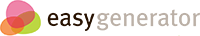# Maths Fun (Percentages)

## Percentages: you'll find out what a percent is, and learn how to calculate percentages.

### Percentages calculation (a simple trick)

#### Whenever you have to calculate percentages you need to know from which number it is being calculated. You can do that easily by filling in a simple table. Let's have a look at the example.Suppose you won the main prize in a lottery. The prize is 100.000. The tax for this prize is 25% and it is already paid by the lottery. So the 100.000 is left after the tax is paid. What is the original prize (including the tax)?Start by filling in the numbers and percentages you know like this:   Number Percentage Prize minus tax 100.000   Tax   25% Original prize includin tax     The trick is to determine where 25% is calculated from. In this case it is 25% from the original prize. That means that the original prize is 100%. Fill this in.   Number Percentage Prize minus tax 100.000   Tax   25% Original prize includin tax   100% This means, the prize minus the tax is 100% minus 25% tax = 75%   Number Percentage Prize minus tax 100.000 75% Tax   25% Original prize includin tax   100% That means 75% equals 100.000. You can now calculate 1% (100.000/75) and if you multiply that by 100 you have 100% (133.333.33). So the tax is 33.333.33.   Number Percentage Prize minus tax 100.000 75% Tax 33.3333.33 25% Original prize includin tax 133.333.33 100% This trick always works. Just make sure you determine the original amount and make it 100%.

Try this now by yourself for the next calculation:

You can buy a shirt for \$ 10. It has a 50% discount. What is the original price (without the discount) ?

Fill in this table:

 Number Percentage Price (with discount) Discount Original price (withou discount)

### Class elections

There are 25 students in Ms. Simposn's first-grade class. In the class election for a head student, 4 students voted for Jack, 9 voted for Tom, and 12 voted for Jane. What percentage of the class voted for each student?

Drag the percentages to the corresponding names of the candidates.

• 36%
• 48%
• 16%
•### What's wrong with the apples?25% of 200 apples are bad. How many apples are bad?

••••